• 字符串二维数组传参
#include<stdio.h>
#include<string.h>
int set(char* (*a), int num)
{
int i=0;
for(int i = 0; i< num; i++)
{
printf("key = %s, value = %s\n", *(a[i]), *(a[i]+1));
}
return 0;
}

int main()
{
int num = 3;
char* sendbuf[num];
sendbuf = "Year";
sendbuf = "2016";
sendbuf = "Month";
sendbuf = "11";
sendbuf = "Day";
sendbuf = "30";
set(sendbuf, num);
return 0;
}

输出：
key = Year, value = 2016
key = Month, value = 11
key = Day, value = 30


展开全文char
• 当我初始化一个字符串二维数组后，输出了在命令窗口后，如果另一个函数改变了其中一个元素的数据，怎么样在原有输出的结果之下，改掉其中的那个元素呢？？？c++ c语言 开发语言
• 利用冒泡排序对字符串二维数组进行排序 package lan; public class array { public static void main(String[] args) { String arr[][]= {{"青年节","5.4"}, {"教师节","9.10"}, {"女生节","3.9"}, {"妇女...

利用冒泡排序对字符串二维数组进行排序

package lan;

public class array {
public static void main(String[] args) {
String arr[][]=
{{"青年节","5.4"},
{"教师节","9.10"},
{"女生节","3.9"},
{"妇女节","3.8"},
{"国庆节","10.1"},
{"元旦","1.1"},
{"劳动节","5.1"},
{"儿童节","6.1"}};
/*
* 通过循环 获取到每个一维数组下标为1的元素
* 通过Double.parseDouble把获取到的字符串数字转为double数
* 通过冒泡排序进行排序
*
* */
System.out.println("排序前的数组:");
printArr(arr);

for(int i=0;i<arr.length-1;i++){
for(int j=0;j<arr.length-1-i;j++){

if(Double.parseDouble(arr[j])>Double.parseDouble(arr[j+1])){
String temp=arr[j];
arr[j]=arr[j+1];
arr[j+1]=temp;
}
}

}

//调用打印方法
System.out.println("排序后的数组:");
printArr(arr);

}
public static void printArr(String[][] arr){
for(int a=0;a<arr.length;a++){
for(int b=0;b<arr[a].length;b++) {
System.out.print(arr[a][b]+"\t");
}
System.out.println();//换行
}
}

}



展开全文java 排序算法
• sizeof 和 strlen 在求解数组 字符数组 字符串 二维数组时候的小结
一维数组
int a[] = {1,2,3,4};
printf("%d\n",sizeof(a));
printf("%d\n",sizeof(a+0));
printf("%d\n",sizeof(*a));
printf("%d\n",sizeof(a+1));
printf("%d\n",sizeof(a));
printf("%d\n",sizeof(&a));
printf("%d\n",sizeof(&a+1));
printf("%d\n",sizeof(&a));
printf("%d\n",sizeof(&a+1));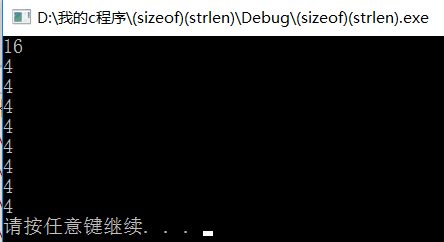解释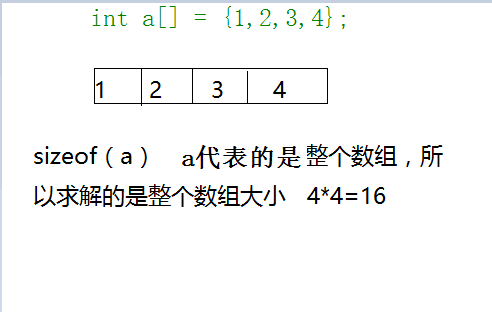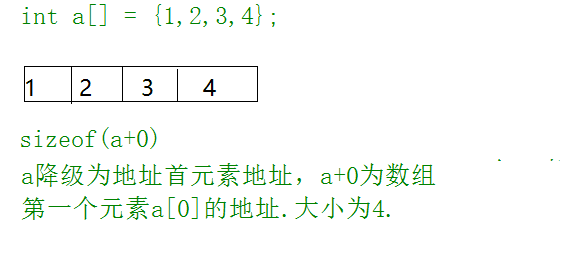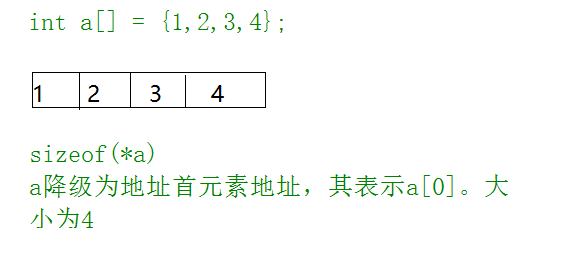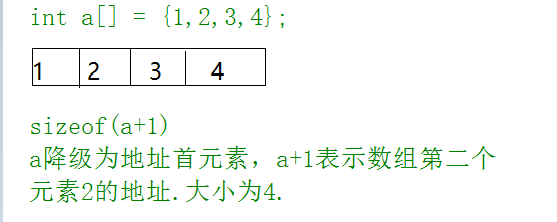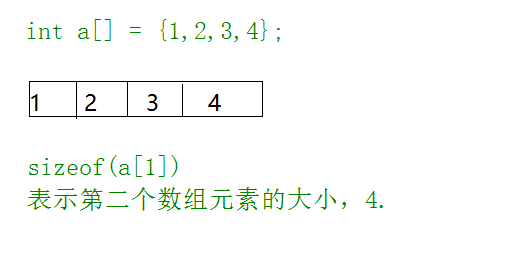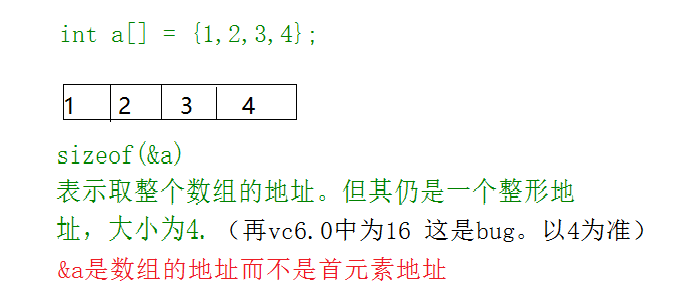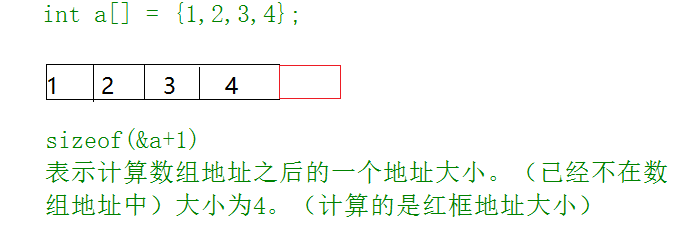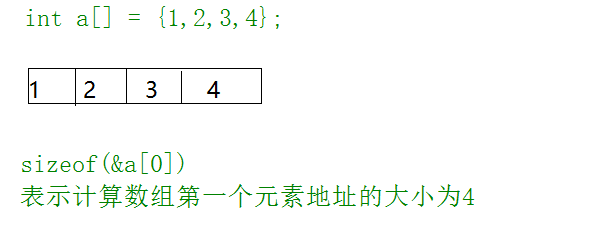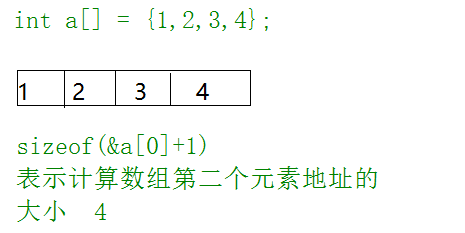字符数组
char arr[] = {'a','b','c','d','e','f'};
printf("%d\n", sizeof(arr));
printf("%d\n", sizeof(arr+0));
printf("%d\n", sizeof(*arr));
printf("%d\n", sizeof(arr));
printf("%d\n", sizeof(&arr));
printf("%d\n", sizeof(&arr+1));
printf("%d\n", sizeof(&arr+1));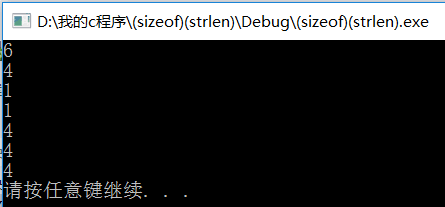解释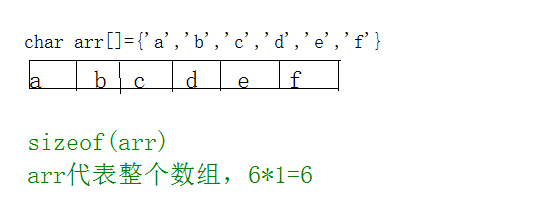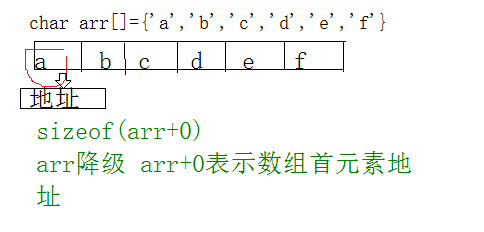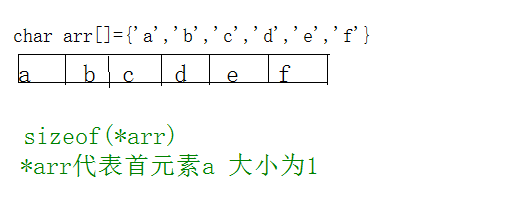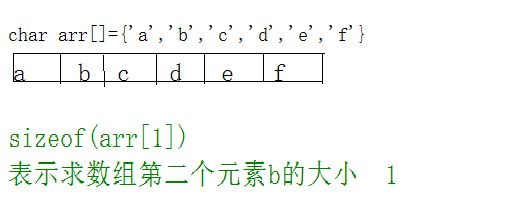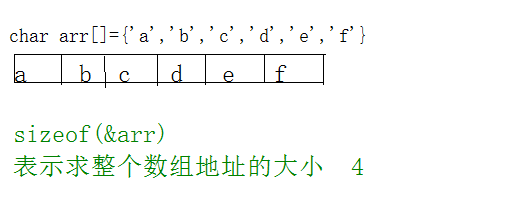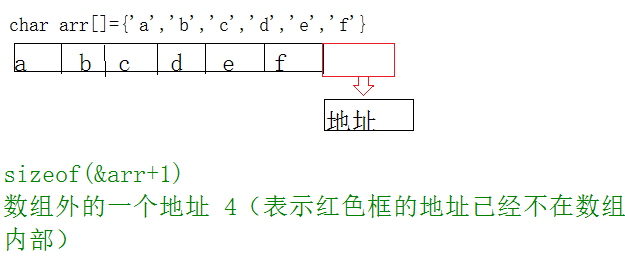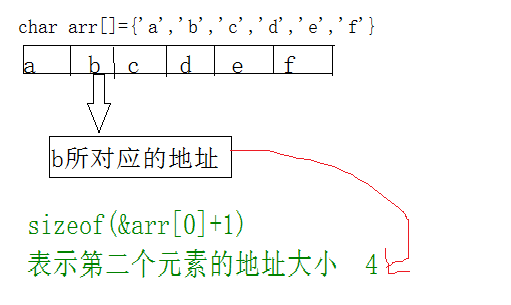char arr[] = {'a','b','c','d','e','f'};
printf("%d\n", strlen(arr));
printf("%d\n", strlen(arr+0));
printf("%d\n", strlen(*arr));
printf("%d\n", strlen(arr));
printf("%d\n", strlen(&arr));
printf("%d\n", strlen(&arr+1));
printf("%d\n", strlen(&arr+1));
这其中有的包含错误 不能编译运行 所以一个个解析
char arr[] = {'a','b','c','d','e','f'};
1 printf("%d\n", strlen(arr));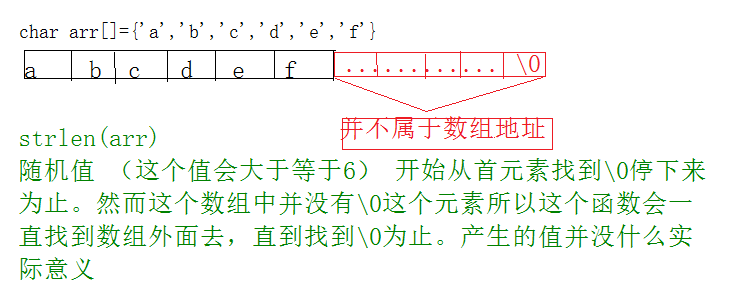2 printf("%d\n", strlen(arr+0));
与上图（1）一样  括号中的arr与arr+0  都表示从第一个元素找起 直到\0结束。同一个系统下产生的随机值也与一样。
3printf("%d\n", strlen(*arr));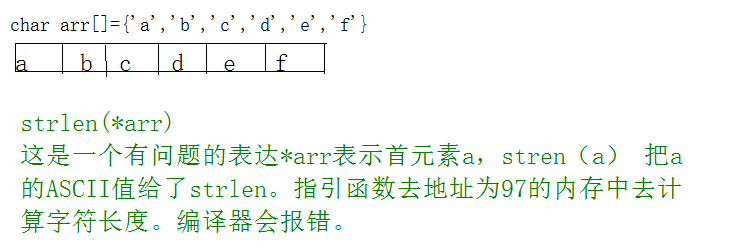4printf("%d\n", strlen(arr));
和3 一个道理  会把b的ASCII值98給strlen然后去寻找   报错
类型不兼容  道理和1.2一样。产生的结果也会和1.2一样
5printf("%d\n",
strlen(&arr));
与1
2 一样
6printf("%d\n",
strlen(&arr+1));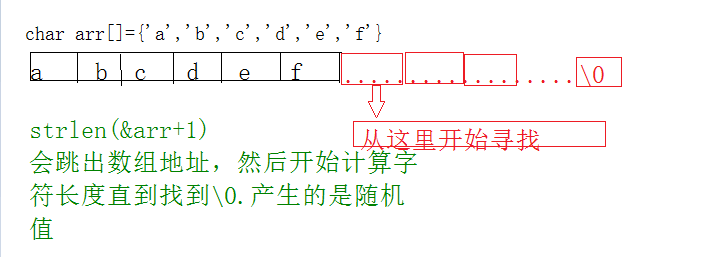printf("%d\n",
strlen(&arr+1));
与1
2 一样   但会比1 2 大小减一   因为在第二个元素开始寻找
字符串：
char
*p = "abcdef";
printf("%d\n",
sizeof(p));
printf("%d\n",
sizeof(p+1));
printf("%d\n",
sizeof(*p));
printf("%d\n",
sizeof(p));
printf("%d\n",
sizeof(&p));
printf("%d\n",
sizeof(&p+1));
printf("%d\n",
sizeof(&p+1));

printf("%d\n", strlen(p));
printf("%d\n",
strlen(p+1));
printf("%d\n",
strlen(*p));
printf("%d\n",
strlen(p));
printf("%d\n",
strlen(&p));
printf("%d\n",
strlen(&p+1));
printf("%d\n",
strlen(&p+1));
解释

char *p = "abcdef";
printf("%d\n", sizeof(p));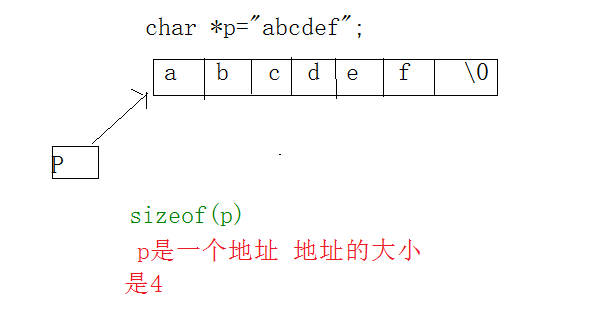printf("%d\n", sizeof(p+1));
与第一个道理一样 p+1表示第二个元素b的地址大小 4
printf("%d\n", sizeof(*p));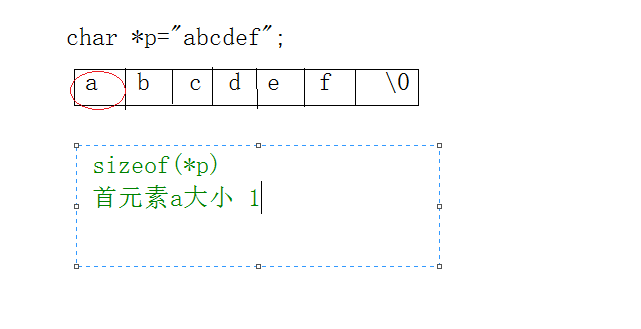printf("%d\n", sizeof(p));
与第三个一样  首元素a的大小 1
printf("%d\n", sizeof(&p));
与&arr一个道理 求得的是整个数组的地址大小4
printf("%d\n", sizeof(&p+1));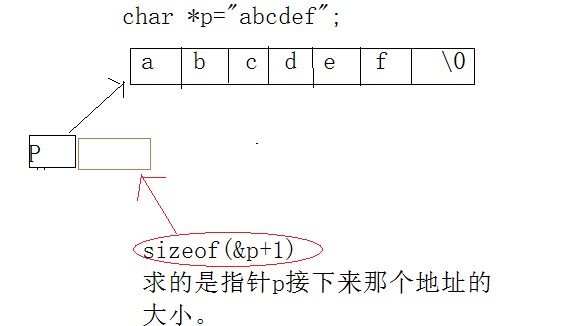printf("%d\n", sizeof(&p+1));
求的是第二个元素的地址大小4

printf("%d\n", strlen(p));
从首元素开始计算字符个数  直到\0结束  （\0不计算在内）
printf("%d\n", strlen(p+1));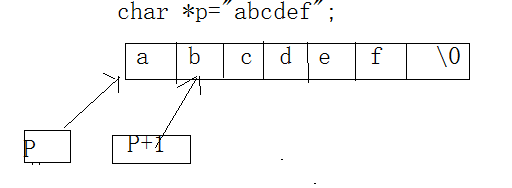printf("%d\n", strlen(*p));
错误的  把a的ASCII码给了函数 去当作地址寻找
printf("%d\n", strlen(p));
同上
printf("%d\n", strlen(&p));
把指针的地址給strlen去寻找  类似下图
printf("%d\n", strlen(&p+1));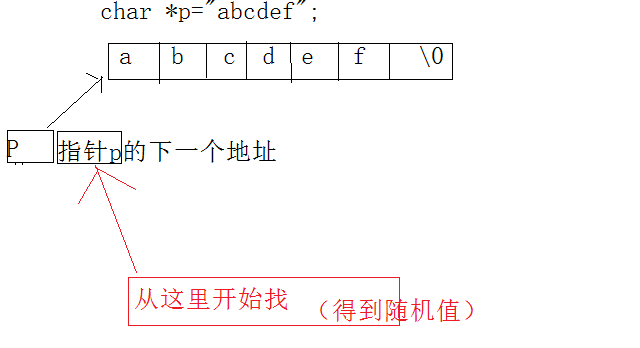printf("%d\n",
strlen(&p+1));
从第二个元素开始找
得到5
二维数组：
int
a = {0};

printf("%d\n",sizeof(a));
printf("%d\n",sizeof(a));
printf("%d\n",sizeof(a));
printf("%d\n",sizeof(a+1));
printf("%d\n",sizeof(a+1));
printf("%d\n",sizeof(&a+1));
printf("%d\n",sizeof(*a));
printf("%d\n",sizeof(a));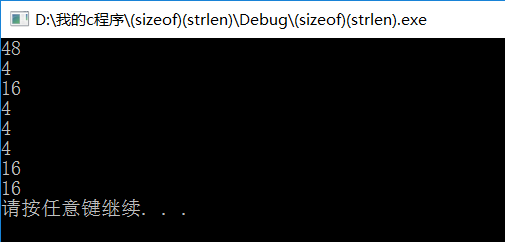解释

int a = {0};

printf("%d\n",sizeof(a));
a表示整个数组12个元素   大小为   12*4=48
printf("%d\n",sizeof(a));
第一行第一列这个元素的大小 4
printf("%d\n",sizeof(a));
a表示第行四个元素  4*4=16
printf("%d\n",sizeof(a+1));
a表示第一行  +1  第一行第二个元素 大小为4
printf("%d\n",sizeof(a+1));
第二行元素的地址  大小为4
printf("%d\n",sizeof(&a+1));
第一行 第二个元素的地址  4
printf("%d\n",sizeof(*a));
表示第一行元素  4*=16  （跟一维数组不同）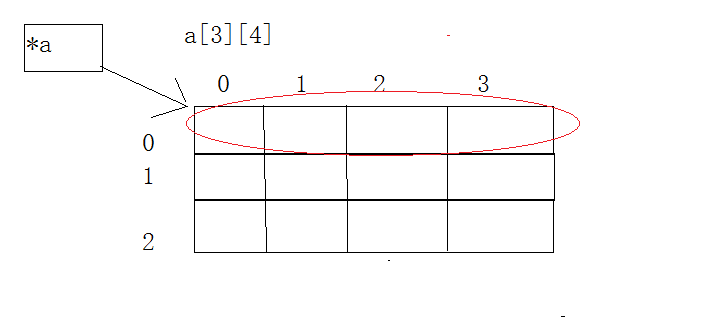printf("%d\n",sizeof(a));
已经跳出数组但地址空间依旧是4个  大小为4*4=16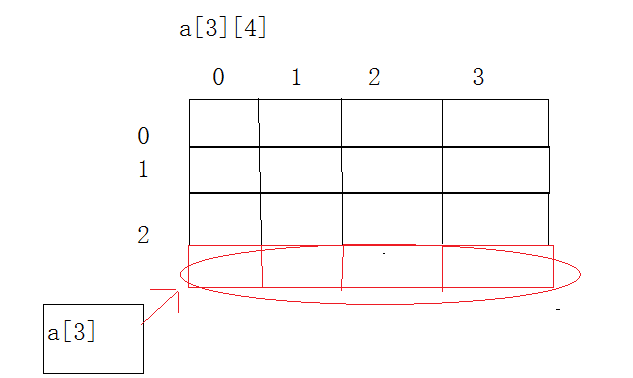展开全文• 我定义了一个动态字符串二维数组如下: //定义条件二维动态数组 typedef String TJsxT; TJsxT *TJsx; TJsx=new TJsxT[TJnum+1]; 这种二维的行与列的理解正确的是:TJsx[TJnum+1]，至于为什么，我个人是...
我定义了一个动态字符串二维数组如下:

//定义条件二维动态数组
typedef String TJsxT;
TJsxT *TJsx;
TJsx=new TJsxT[TJnum+1];

这种二维的行与列的理解正确的是:TJsx[TJnum+1]，至于为什么，我个人是这样理解的，实际测试中就是这样理解的，反之错误。从定义上来讲，应当是先你申请了一个TJsxT这样的一个类，所以只能这样理解了。

展开全文• 如何使用指针访问二维数组？ 先来看看在一维数组中怎样使用指针访问数组： 一维数组中 a[i] 中的 a 代表了本数组的首地址，相当于 &a。 因此 *a 就等于 a。 那么对 a 加 1，就可以访问下一位： *(a+1) 就...
• 文章目录POI5.0将Excel之xlsx转为String[][]字符串二维数组简单试用一、POI是什么？二、使用步骤1.Maven引入2.示例代码总结 POI5.0将Excel之xlsx转为String[][]字符串二维数组简单试用 一、POI是什么？ Apache ...java excel poi
• c++字符串二维数组解析|std::string::find_first_of
• ctype.h：包括：isalpha() ;idigit() ; isprint() ;... mg[ ]={" " , "######", "%%%%%%%%%"} 这是字符串二维数组。mg【】的每一个元素都是一个字符串。 书上最后输出的时候用了数学公式。鬼畜，...
• 现在从txt文档中读取一个矩阵，把矩阵读取完毕了，但是是一个string二维数组，我的矩阵是228*228的，比较大 现在想把string转化成int二维数组，请问怎么做才能实现，本人小白，还请详解，谢谢[img=...
• 该方式是可以直接使用的，主要用于字符串数组作为形参传入函数时使用： void csdfa(char (*aaa)) { rt_strncpy(aaa,"nv_sfme... //申请一个 4行 5列的二维数组 char (*ar)[col] = (char(*)[col])malloc(sizeofc语言 指针 malloc
• 输入一个字符串，以回车结束（字符串长度<=100）。该字符串由若干个单词组成，单词之间用一个空格隔开，所有单词区分大小写。现需要将其中的某个单词替换成另一个单词，并输出替换之后的字符串。 输入 多组数据...
• “福到了” 字符串二维数组+打印+getlline()+getchar() #include<iostream> #include<cstdio> #include<string> using namespace std; string a; int main() { int i, j, k, n; char ch...
• 比如了定义如下函数和字符串二维数组，如何定义一个函数，把二维数组当做形参传递进去？ #include<cstring> #include<iostream> #include<stdio.h> #include<stdlib.h> using ...
• 最先想到的是尝试所有N！种交换方式,但是时间复杂度不行 package 数字技巧实战; import java.util.Scanner; ...public class CrazyRows { ... public static void main(String[] args) { // TODO Auto-generated ...
• #include<stdio.h>...//对一个字符串二维数组进行操作，必须赋予指针吗？比如该题，为什么不能传送ch?反正都是地址。 void stsrt(char * string[],int num) { ********* }//麻烦讲解一下。
• 在java中运行这一句代码: String[][] str = new String; 假设是第一次运行,在内存中会产生多少个对象?请说明理由,不要说一个单纯的数字.谢谢.java 对象
• struct node { char data; } s; bool cmp(const node &x, const node &y) { if(strcmp(x.data, y.data) 0) return true; return false; } sort(s, s+n, cmp);
• 字符串数组：比如 char a={"hello","goodbye","goodb"}; 如果想把此字符串数组传出去 void func(char (*mat)); 版权声明：本文为博主原创文章，遵循 CC 4.0 by-sa 版权协议，转载请附上原文出处链接...
• 今天根据业务需要，使用Python调用C++编写的so，调用相关接口获取部分信息，实现过程大致如下： C/C++端： /**  *获取信息（分配内存并赋值） */ extern "C" __declspec(dllexport) void getInfo(char*...
• 直接上代码：#includevoid Fun(int *a[],int m,int n)//{printf("%d\t",*a);///*int e={8,7,6,5,4,3,2,1};int *f,***g;...*/}/*解释：Fun()中的int *a表示定义指针数组a，a,a...
• 如题 这样是不对的吧： string[][] s = new string;
• //编写一个函数：从传入的num个字符中找到最长的一个字符，并通过max传回该地址。 //重点：切记这里a就是一个地址。 1 #include<conio.h> 2 #include<stdio.h> 3 #include<string.h>...
• 本文探讨在MeeGo平台上通常会并存的两种DBUS绑定：DBUS－GLIB Binding与QT DBUS Binding之间的传递"aas（字符串二维数组）“ 之间的问题。本文的讨论以一个使用DBUS－GLIB的绑定DBUS的服务器端和一个使用QT DBUS ...qt binding service 服务器...